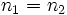# Equality of left and right nil element

Jump to: navigation, search
This article gives a statement (possibly with proof) of how, if a left-based construction and a right-based construction both exist, they must be equal.
View other such statements

## Statement

Suppose$(S,*)$ is a magma (a set$S$ with a binary operation$*$). Suppose$n_1$ is a left nil element (i.e.,$n_1 * a = n_1$ for all$a \in S$) and$n_2$ is a right nil element for$S$ (i.e.,$a * n_2 = n_2$ for all$a \in S$). Then,$n_1 = n_2$.

## Proof

### Proof idea

A left nil element can be thought of as an element that dominates the product when placed on the left, and a right nil element is an element that dominates the product when placed on the right. To show that these are equal, we need to pit the left and right nil elements against each other. Since both of them must dominate, they must both be equal.

### Formal proof

Given: A magma$S$ with binary operation$*$, a left nil element$n_1$ for$*$, a right nil element$n_2$ for$*$.

To prove:$n_1 = n_2$

Proof: Consider the product$n_1 * n_2$. Since$n_1$ is a left nil element,$n_1 * n_2 = n_1$. Since$n_2$ is a right nil element,$n_1 * n_2 = n_2$. Thus,$n_1 = n_2$.